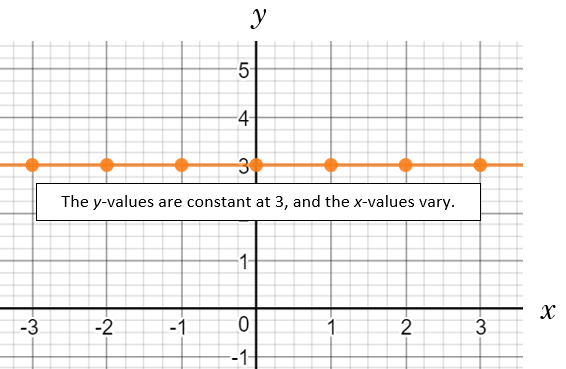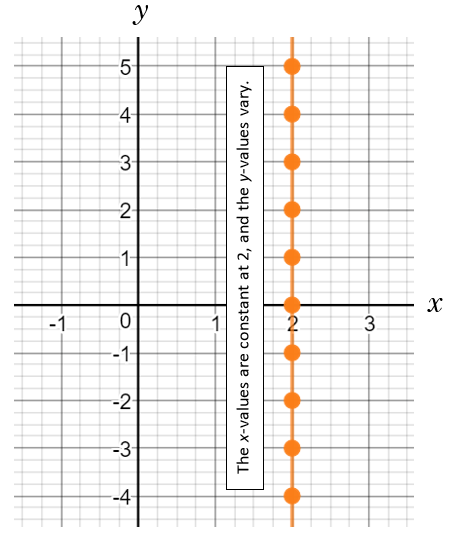# LINR 1 | Lesson 3 | Making Connections (Increase or Decrease)# Increase or Decrease

 A line with a positive slope points up to the right.A line with a negative slope points down to the right.A linear function is increasing when the $$x$$-values increase and the $$y$$-values increase.Created using Desmos.com This line is increasing. An increasing line has a positive slope $$(m>0)$$. A linear function is decreasing when the $$x$$-values increase and the $$y$$-values decrease.Created using Desmos.com This line is decreasing. A decreasing line has a negative slope $$(m<0)$$. A linear function is constant when the $$x$$-values increase and the $$y$$-values remain the same.This line is a horizontal line. A horizontal line has zero slope $$(m=0)$$. A linear function is undefined if the $$x$$-values are constant as the $$y$$-values vary.This line is a vertical line. A vertical line has undefined slope.